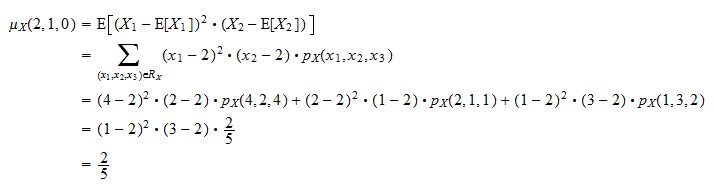StatLect

# Cross-moments of a random vector

This lecture defines the notion of cross-moment of a random vector, which is a generalization of the concept of moment of a random variable (see the lecture entitled Moments of a random variable).## Cross-moment

Letbe arandom vector. A cross-moment ofis the expected value of the product of integer powers of the entries of:whereis the-th entry ofandare non-negative integers.

The following is a formal definition of cross-moment.

Definition Letbe arandom vector. Letand. If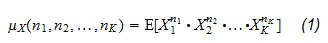exists and is finite, then it is called a cross-moment ofof order. If all cross-moments of orderexist and are finite, i.e. if (1) exists and is finite for all-tuples of non-negative integerssuch that, thenis said to possess finite cross-moments of order.

The following example shows how to compute a cross-moment of a discrete random vector.

Example Letbe adiscrete random vector and denote its components by,and. Let the support ofbe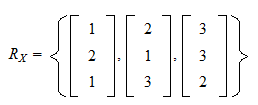and its joint probability mass function be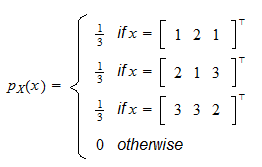The following is a cross-moment ofof order:which can be computed by using the transformation theorem: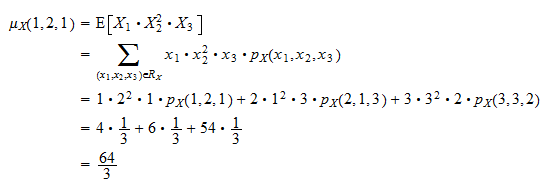## Central cross-moment

The central cross-moments of a random vectorare just the cross-moments of the random vector of deviations.

Definition Letbe arandom vector. Letand. If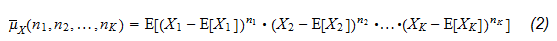exists and is finite, then it is called a central cross-moment ofof order. If all central cross-moments of orderexist and are finite, that is, if (2) exists and is finite for all-tuples of non-negative integerssuch that, thenis said to possess finite central cross-moments of order.

The following example shows how to compute a central cross-moment of a discrete random vector.

Example Letbe adiscrete random vector and denote its components by,and. Let the support ofbe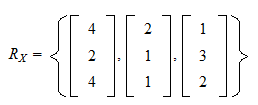and its joint probability mass function be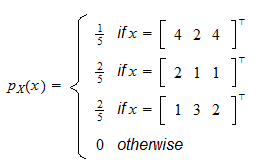The expected values of the three components ofareThe following is a central cross-moment ofof order: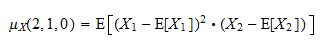which can be computed by using the transformation theorem: